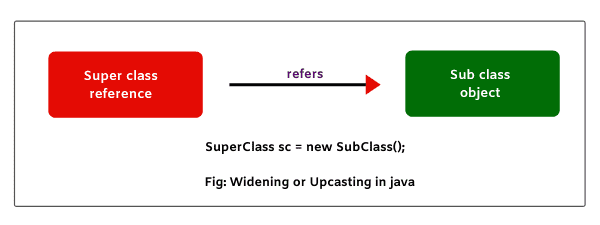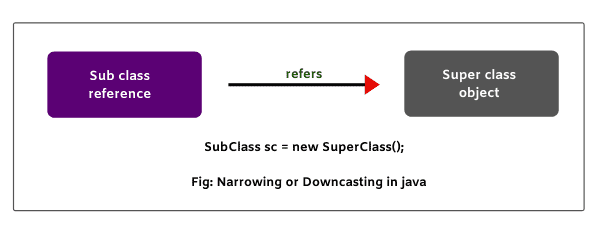# Upcasting and Downcasting in Java with Example

## What is Upcasting (Widening)

When the reference variable of super class refers to the object of sub class, it is known as widening or Upcasting in java

In other words, when sub class object type is converted into super class, it is called widening or upcasting.Let’s understand it with a suitable example. Suppose class One and class Two are related classes through inheritance. class One is a super class and class Two is sub class of One.

1. Super class reference is used to refer to super class object.
One o = new One();
In the above statement, class One’s reference o is pointing or referring to One’s object.

2. Similarly, sub class reference is used to point to sub class object.
Two t = new Two();
In this statement, class Two’s reference t is pointing to Two’s object.

In the above two statements, we do not need casting. This is because on the left side and at right side of assignment operator (=), we have the same data type. For example, in the first statement, o is reference of class One. So, data type of o is One.

At right side of assignment operator, we have class One’s object. Data type of the class object is also One. Therefore, casting is not required in this case.

Till here, there is no need for casting. But when a reference of class refers to different class’s object, we need casting.
For example:
One o = new Two(); // class One’s reference o is pointing to class Two’s object.
In this statement, on the left side, the data type of reference o is One but on the right side, we got object whose data type is Two. So, in this case, we will need casting.

We will convert object’s data type into class O using cast operator. It can be done like this:
One o = (One) new Two(); // Converting class Two’s type into class One.

Here, sub class object type has been converted into super class type. This kind of conversion is called upcasting or widening in java. If we do not use cast operator in the above case, even we will not get any error message. Java compiler will do implicit casting.

Let’s take an example program to see the effect of upcasting where super class reference refers to sub class object.
Program source code 1:

```package classCasting;
public class One
{
void m1()
{
System.out.println("m1 method in class One");
}
}
public class Two extends One
{
void m2()
{
System.out.println("m2 method in class Two");
}
}
public class Test
{
public static void main(String[] args)
{
One o = (One)new Two(); // Upcasting. Here, super class reference o refers to sub class object.
o.m1();
// o.m2(); // Compile-time error message.
}
}```
```Output:
m1 method in class One```

In the above program, super class reference is pointing to sub class object. This means that sub class object type is converted into super class type. Now, observe that we are able to call m1 method of super class.

But when we are trying to call m2 method of sub class, we got error message: compile-time error. Hence, in upcasting or widening, we can call all methods of super class but not sub class methods.

Let’s take another program where we will override super class method in sub class and will observe that can we access super class method and sub class method.

Program source code 2:

```package classCasting;
public class One
{
void m1()
{
System.out.println("m1 method in class One");
}
}
public class Two extends One
{
void m1()
{
System.out.println("m1 method in class Two");
}
}
public class Test
{
public static void main(String[] args)
{
One o = (One)new Two(); // Upcasting. Here, super class reference o refers to sub class object.
o.m1();
}
}```
```Output:
m1 method in class Two```

As you can observe that it is possible to access sub class method but not super class method when super class method is overridden in sub class. This shows that you can access only 50% of functionality.

## Downcasting (Narrowing) in Java

When subclass reference refers to super class object, it is called narrowing or downcasting in java. In other words, when sub class type is converted into super class type, it is called downcasting.For example:
Two t = (Two) new One();
// Here, we are converting super class object type into sub class type. Therefore, we need a compulsory cast operator. If we perform it directly, Java compiler will give compile-time error.

Let’s take the above example program where we will do downcasting and see what happens?
Program source code 3:

```package classCasting;
public class One
{
void m1()
{
System.out.println("m1 method in class One");
}
}
public class Two extends One
{
void m2()
{
System.out.println("m2 method in class Two");
}
}
public class Test
{
public static void main(String[] args)
{
Two t = (Two) new One(); // Downcasting. Here, sub class reference t refers to super class object.
t.m1();
t.m2();
}
}```
```Output:
Exception in thread "main" java.lang.ClassCastException: classCasting.One cannot be cast to classCasting.Two```

In the above program, we have performed downcasting directly, therefore, JVM thrown ClassCastException at run time. So, in the case of downcasting, we cannot access any of methods of super class or sub class. So, let’s see how downcasting is possible in java?

## How Downcasting is possible in Java?

To overcome this problem, we will have to modify in the previous code. So, let’s see the following source code.
Program source code 4:

```package classCasting;
public class One
{
void m1()
{
System.out.println("m1 method in class One");
}
}
public class Two extends One
{
void m2()
{
System.out.println("m2 method in class Two");
}
}
public class Test
{
public static void main(String[] args)
{
One o = new Two(); // Super class reference refers to sub class object.
Two t = (Two)o; // Converting super class reference type into sub class reference type.
t.m1(); // Calling m1 method using reference variable of sub class.
t.m2(); // Calling m1 method using reference variable of sub class.
}
}```
```Output:
m1 method in class One
m2 method in class Two```

In the above program, super class reference type has been converted into sub class reference type. Now, we are able to call both methods m1() and m2() using reference variable of sub class.

In the preceding code, you can observe that if we create an object of sub class, it is possible to access all the methods of super class as well as sub class. In this way, downcasting is possible in java.

Final words
Hope that this tutorial has covered almost all important points related to upcasting and downcasting in java with example program. I hope that you will have understood this topic nicely and enjoyed related programming.